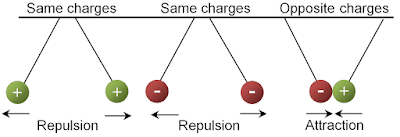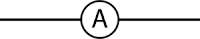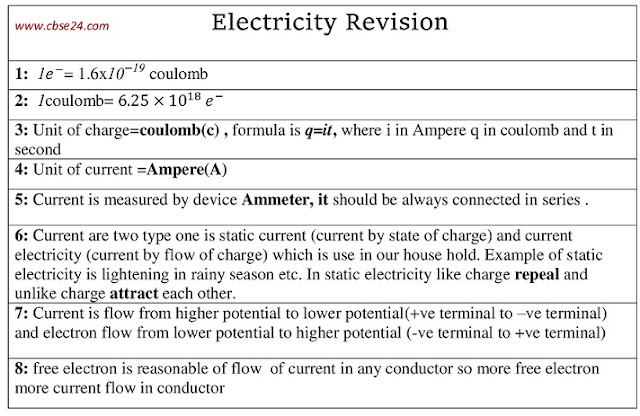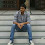# Electricity class 10 notes | cbse24

Electricity (Branch of physics). Electricity is an important source of energy in modern times
Electricity can be classified into two parts

1:Static electricity 2:Current electricity

Note: Before going to start static and current electricity, we first understand what is charge?

Electric charge:- Electric charge is an intrinsic property of the elementary particles like electrons, protons, etc. of which all the objects are made up of, it is because of these electric charges that various objects exert a strong electric force of attraction or repulsion on each other.

Example: Suppose we take a wire made of copper, so atomic number of copper atom is Z=29,so Z=number of proton(+e)=no of electron(-e)=29,so in CU 29 proton(+e) and electron(-e) they bound each other tightly so, Net Charge=0.Charge Behaviour

Charge Behaviour:-
• +ve charge & +ve charge→ Re-pale each other
• +ve charge & -ve charge → Attract each other
• -ve charge & -ve charge → Re-pale each other
• -ve charge & +ve charge → Attract each other

Note -Like charge re-pale and unlike charge attract each other
"Now we understand what is charge now we going to understand static & current electricity"

1:-Static Electricity:-(charge remains at rest, they not move)Static electricity is an imbalance of electric charges within or on the surface of a material. The charge remains until it is able to move on away by means of an electric current or electrical discharge.

Example:-Lightning which we see in the sky during the rainy season also involves static electricity

"If potential gradient becomes 10kv/cm in the cloud the air immediately surround the cloud get the ionized and first process of the lightning start, currently associated with this stream is of the order of 100A, this is such a big value of current in our home generally 2 to 6 A current comes so compare its value which is huge, which can damage more, and speed should be 0.1m/µs."

2:Current Electricity:-(use daily in our home)
Q:- First understand, What is current?

Ans:-When we connect a wire with a battery than current flow, so actually we create a potential difference between wire so electric charge starts to flow.Direction of flow of electron and current

• The direction of the electron(-e) is -ve → +ve terminal of battery mean lower potential to higher potential.
• The direction of current (I) is +ve → -ve terminal of battery mean the higher potential to lower potential.
"Current is Scalar quantity, the electric current has magnitude & direction both but It is a scalar quantity(because the law of vector is not applicable, it follow the law of algebraic sum) I=Q/t where: Q in coulomb and t in second"

The SI unit of electric current is⟶ Ampere(A)

(The magnitude of electric current in a conductor is the amount of electric charge passing through a giving point of the conductor in one second or rate of flow of charge)

Q:-How current is measured?

Ans:-With the help of an instrument called Ammeter, current is measure. An ammeter is a low resistance device, should always connect in series not in parallel if we connect this in parallel lots of current flow through it and its blow out.Symbol of ammeter

### How the current flow in a wire

Suppose to take a metal wire of copper in that lots of tiny particles called atom so take a single atom to understand free-electron first, in that no of proton =no of electron =29 so nuclei holds all-electron around us. In outermost only 1ē present which is far distance from the nucleus, so little increase of temperature this electron bounce and become free and this electron is responsible for current flowing in a wire.Picture of free electron from atom

Metal has a large number of free electron,/cubic. Meter In the absence of any electric field, these electrons are in a state of continuous random motion due to thermal energy. At room temperature, they move with velocities of the order of m/s. However, these velocities are distributed randomly in all directions. There is no perfect direction of motion. If U1, U2...Un  is the random velocities of n free electron, then the average velocity of the electron will be zero. You can see in figure 1(a

Thus there is no net flow of charge in any direction. In the presence of an external field in any direction, experience a force(F= -eE) in the opposite diction of E(since an electron has a negative charge) and electron undergoes an acceleration. You can see in figure 1(b).behaviour of free electron in a wire

### Revision of these  topics### A mixed question of S.Chand and NCERT of this topic.

Q1: Should the resistance of an ammeter be low or high?
Ans: The resistance of an ammeter should below so that it will not disturb the magnitude of the current flowing through the circuit when connected in series in a circuit

Q2: How does the use of a fuse protect electrical appliances?
Ans: The fuse wire is always connected in series with the live wire or electrical devices. If the flow of current exceeds the specified value due to some reason, the heat produced melts it and disconnects the circuit or the device from the mains. In this way, fuse wire protects the electrical appliances.

"Test your knowledge by giving online Exam(quiz)"

Note:-"Click on this link and test your knowledge just simple and basic type of objective quiz which increase your understanding power "
1:-Test1

1.Sir door Wala samj me nhi AA RHA hai

2.3.Very good website

4.Karin ke leye

5.1.Thanku so much...

2.Very nice

6.Nice website sir ☺

7.Thank you so much sir

8.Mst website

9.Nice website sir

10.11.12.13.I propose merely very good along with reputable data, consequently visualize it: kündigung

Previous Post Next Post